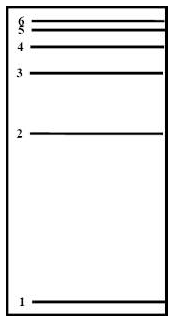# Problem: Which transition would result in the emission of photons with the lowest frequency?

###### FREE Expert Solution

Recall that starting from n = 1, the distance between each energy level gets smaller as shown below:Emission is a transition process from a higher energy level to a lower energy level

Absorption is a transition process from a lower energy level to a higher energy level

Recall that the energy of a photon is given by:We can see that energy and frequency are directly proportional. The energy in a transition also depends on the distance between the energy levels.

96% (182 ratings)###### Problem DetailsWhich transition would result in the emission of photons with the lowest frequency?

Frequently Asked Questions

What scientific concept do you need to know in order to solve this problem?

Our tutors have indicated that to solve this problem you will need to apply the Bohr Model concept. You can view video lessons to learn Bohr Model. Or if you need more Bohr Model practice, you can also practice Bohr Model practice problems.